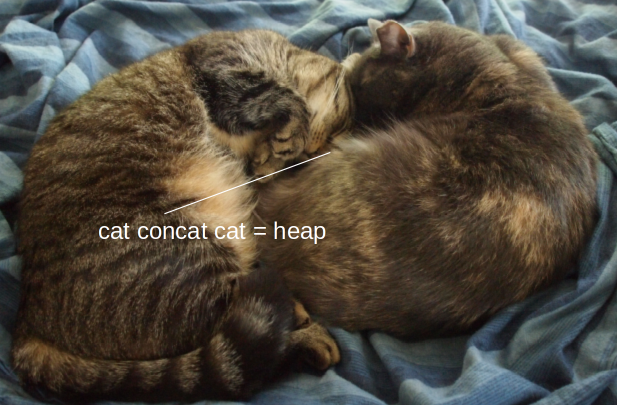CSE-250 Fall 2022 - Section B - Priority Queues, HeapsOct 24, 2022

## Priority Queues

#### PriorityQueue[A <: Ordering]

enqueue(v: A): Unit
Insert a value $v$ into the priority queue.
dequeue: A
Remove the greatest element in the priority queue.
Peek at the greatest element in the priority queue.

#### Priority Queues

Operation Lazy Proactive
enqueue $O(1)$ $O(n)$
dequeue $O(n)$ $O(1)$
head $O(n)$ $O(1)$

Can we do better?

#### Priority Queues

Lazy
Fast Enqueue, Slow Dequeue
Proactive
Slow Enqueue, Fast Dequeue
???
Fast(-ish) Enqueue, Fast(-ish) Dequeue

Idea: Keep the priority queue "kinda" sorted.

Larger items tend to be closer to the front of the list. The closer we are to the front of the list, the more sorted it gets.

#### Binary Heaps

Challenge: How do we keep track of which elements are still sorted?

#### Binary Heaps

Idea: Organize the priority queue as a tree

Directed: A directed edge means "≥"

#### Trees

Child
An adjacent node connected by an out-edge
Leaf
A node with no children
Depth of a node
The number of edges from the root to the node
Depth of a tree
The maximum depth of any node in the tree
Level of a node
The depth + 1

#### Binary Heaps

Organize the priority queue as a tree

Directed: A directed edge means "≥"

Binary: Max out-degree of 2 (Easy to reason about)

Complete: Every "level" except the last is full

• Balanced: TBD (loosely, all leaves at ~ same level)
• Easy to encode in an array (later today)

All nodes in the last level are all-the-way left

Ordering by "≥" gets us a "Max-Heap"

#### Heaps

What is the depth of a binary heap containing $n$ items?

• Level 1: Up to 1 item
• Level 2: Up to 2 items
• Level 3: Up to 4 items
• Level 4: Up to 8 items
• Level 5: Up to 16 items
• Level $i$: Up to $O(2^i)$ items

#### Heaps

What is the depth of a binary heap containing $n$ items?

$$n = O\left(\sum_{i = 1}^{\ell_{max}} 2^i\right) = O\left(2^{\ell_{max}}\right)$$

$$\ell_{max} = O\left(\log(n)\right)$$

enqueue(elem: A) [pushHeap]
Place an item into the heap
dequeue: A [popHeap]
Remove and return the maximal element from the heap
The maximal element in the heap
length: Int
The number of elements in the heap.

#### enqueue

Idea: Insert element at next available spot, then fix.

• Call the insertion point current
• While current != root and current > parent
• Swap current with parent
• Repeat with currentparent

#### enqueue


def fixUp[A: Ordering](current: Vertex[A]): Unit =
{
if(current.parent.isDefined){
val parent = current.parent.get
if( Ordering[A].lt( parent.value, current.value ) ){
swap(current.value, parent.value)
fixUp(parent)
}
}
}


What's the complexity?

(How many swaps are required?)

#### dequeue

Idea: Replace root with last element, then fix.

• While current has a child > current
• Swap current with larger child
• Repeat with currentchild

#### enqueue


def fixDown[A: Ordering](current: Vertex[A]): Unit =
{
if(current.leftChild.isDefined){
val left = current.leftChild.get
if( Ordering[A].lt( current.value, left.value ) ){
if(current.rightChild.isDefined){
val right = current.rightChild.get
if( Ordering[A].lt( right.value, left.value ) ){
swap(current.value, left.value); fixDown(left)
} else {
swap(current.value, right.value); fixDown(right)
}
} else {
swap(current.value, left.value); fixDown(left)
}
} else if(current.rightChild.isDefined) {
val right = current.rightChild.get
if( Ordering[A].lt( current.value, right.value ) ){
swap(current.value, right.value); fixDown(right)
}
}
}
}


What's the complexity?

#### Priority Queues

Operation Lazy Proactive Heap
enqueue $O(1)$ $O(n)$ $O(\log(n))$
dequeue $O(n)$ $O(1)$ $O(\log(n))$
head $O(n)$ $O(1)$ $O(1)$

#### Storing Heaps

• Each layer has a maximum size
• Each layer grows left-to-right
• Only the last layer grows

Idea: Use an ArrayBuffer to store the heap.

#### Analysis

Enqueue
Append to ArrayBuffer: $O(n)$, Amortized $O(1)$
fixUp: $O(\log(n))$ fixes, each $O(1)$ = $O(\log(n))$
Total: Amortized $O(\log(n))$, Worst-case $O(n)$
Dequeue
Remove End of ArrayBuffer: $O(1)$
fixDown: $O(\log(n))$ fixes, each $O(1)$ = $O(\log(n))$
Total: Worst-case $O(\log(n))$